# Learning Spark (0) - 总览及第三章结构化API

Databricks 出了本《Learning Spark》的电子书，来学习一下。

## 总览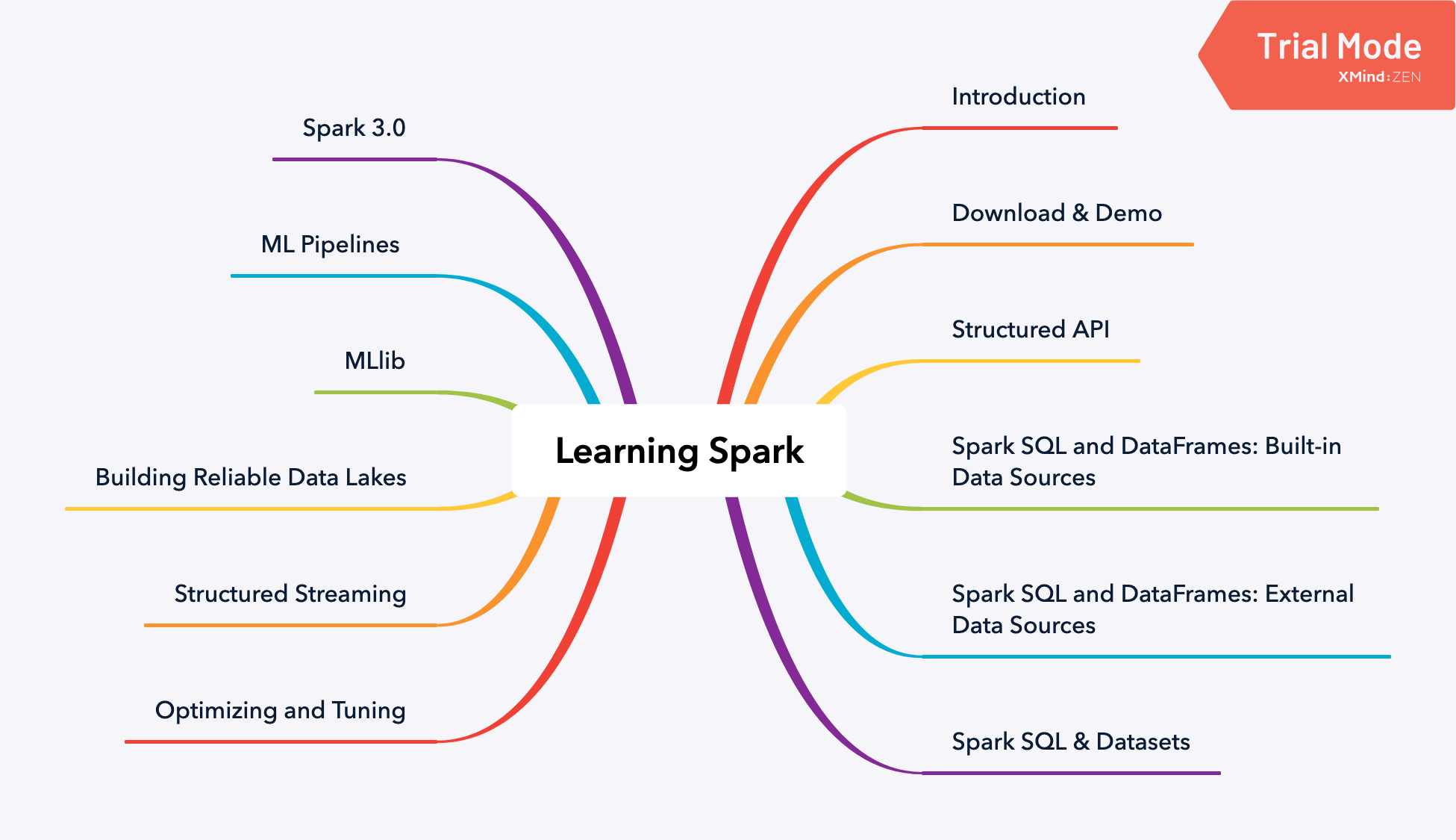1. 基本介绍
2. Spark SQL 使用与调优
3. Streaming
4. MLlib
5. Spark 3.0 的新特性

## RDD 到底是什么？

RDD 是 Spark 最基础的抽象。它分为三部分：

1. Dependencies 依赖
2. Partitions 分区
3. Compute funtion: Partitions => Iterator[T] 计算函数

## 结构化Spark

Spark 2.x 为结构化Spark提供了几个关键方案。

### 主要优点和好处

• expressivity 表现力
• simplicity 简单性
• composability 可组合性
• uniformity 统一性

``````# In Python
# Create an RDD of tuples (name, age)
dataRDD = sc.parallelize([("Brooke", 20), ("Denny", 31), ("Jules", 30), ("TD", 35)， ("Brooke", 25)])
# Use map and reduceByKey transformations with their lambda # expressions to aggregate and then compute average
agesRDD = (dataRDD
.map(lambda x: (x, (x, 1)))
.reduceByKey(lambda x, y: (x + y, x + y))
.map(lambda x: (x, x/x)))
``````

high-level API：

``````# In Python
from pyspark.sql import SparkSession
from pyspark.sql.functions import avg
# Create a DataFrame using SparkSession
spark = (SparkSession
.builder
.appName("AuthorsAges")
.getOrCreate())
# Create a DataFrame
data_df = spark.createDataFrame([("Brooke", 20), ("Denny", 31), ("Jules", 30), ("TD", 35), ("Brooke", 25)], ["name", "age"])
# Group the same names together, aggregate their ages, and compute an average
avg_df = data_df.groupBy("name").agg(avg("age"))
# Show the results of the final execution
avg_df.show()

+------+--------+
|  name|avg(age)|
+------+--------+
|Brooke|    22.5|
| Jules|    30.0|
|    TD|    35.0|
| Denny|    31.0|
+------+--------+
``````

``````// In Scala
import org.apache.spark.sql.functions.avg
import org.apache.spark.sql.SparkSession
// Create a DataFrame using SparkSession
val spark = SparkSession
.builder
.appName("AuthorsAges")
.getOrCreate()
// Create a DataFrame of names and ages
val dataDF = spark.createDataFrame(Seq(("Brooke", 20), ("Brooke", 25), ("Denny", 31), ("Jules", 30), ("TD", 35))).toDF("name", "age")
// Group the same names together, aggregate their ages, and compute an average
// Show the results of the final execution
avgDF.show()
``````

## DataFrame API

### 基本数据类型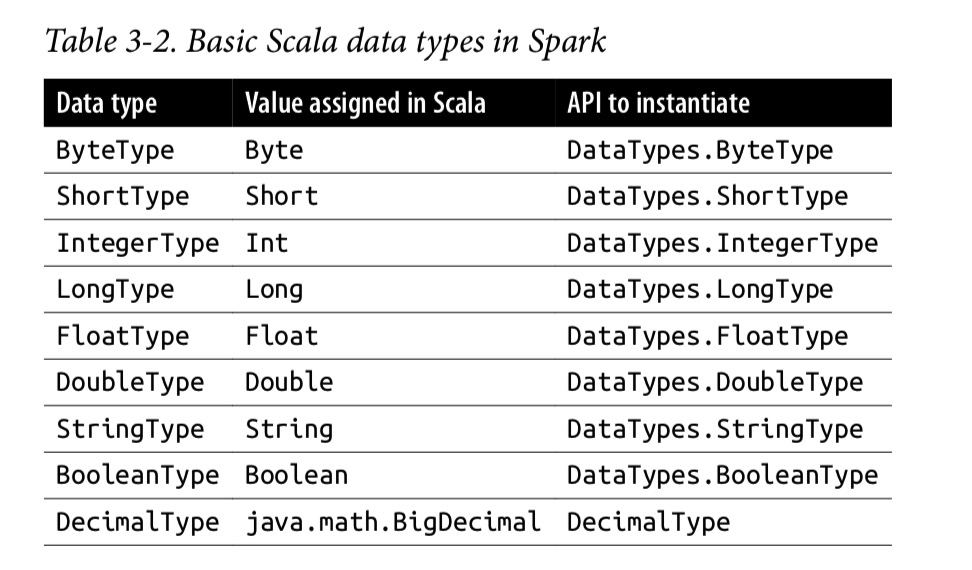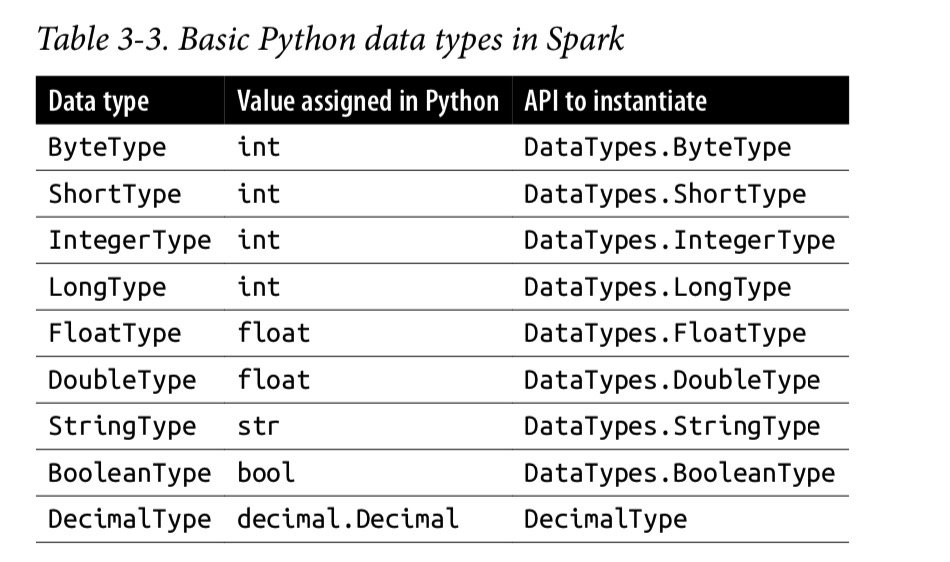### 结构化的和复杂的数据类型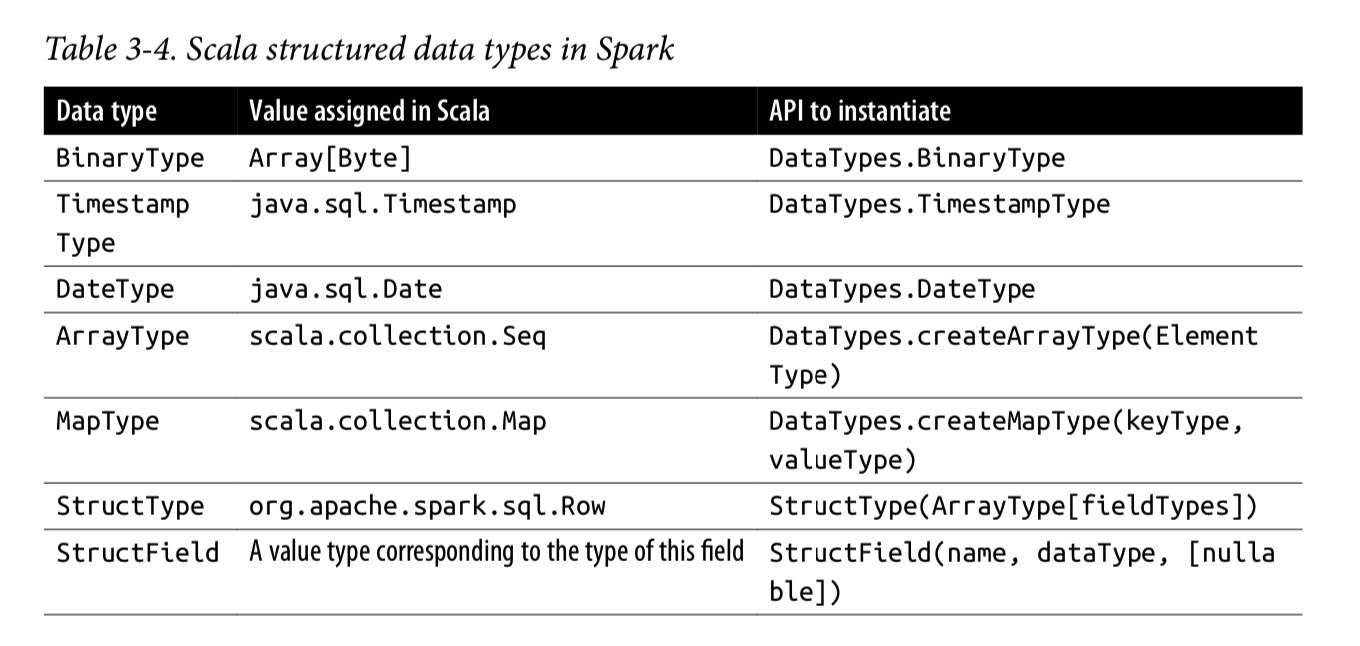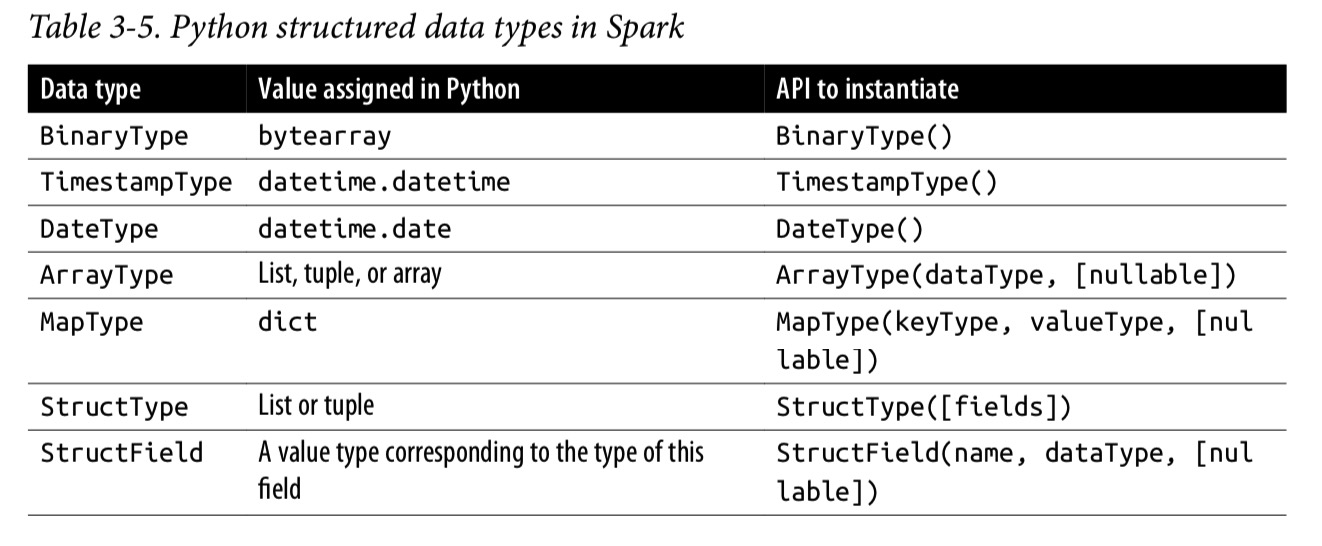### Schemas 和create DataFrame

Spark中的schema定义了DataFrame的列名和关联的数据类型。

• 您可以把 Spark 从推断数据类型的负担中释放出来。
• 您可以阻止Spark创建单独的作业，而只是为了读取文件的大部分内容以确定schema，这对于大型数据文件而言可能既昂贵又费时。
• 您可以及早发现错误，如果数据与架构不匹配。

#### 定义 schema

Using Spark DataFrame API:

``````// In Scala
import org.apache.spark.sql.types._
val schema = StructType(Array(StructField("author", StringType, false),StructField("title", StringType, false), StructField("pages", IntegerType, false)))
``````
``````# In Python
from pyspark.sql.types import *
schema = StructType([StructField("author", StringType(), False),
StructField("title", StringType(), False),
StructField("pages", IntegerType(), False)])
``````

Using DDL:

``````// In Scala
val schema = "author STRING, title STRING, pages INT"

# In Python
schema = "author STRING, title STRING, pages INT"

df = spark.createDataFrame(data, schema)
``````

### 列和表达式

Spark 也支持在column上进行逻辑转换：expr(“columnName * 5”) or (expr(“colum nName - 5”) > col(anothercolumnName)) 。

``````scala> import org.apache.spark.sql.functions._
scala> blogsDF.columns
res2: Array[String] = Array(Campaigns, First, Hits, Id, Last, Published, Url)
// Access a particular column with col and it returns a Column type
scala> blogsDF.col("Id")
res3: org.apache.spark.sql.Column = id
// Use an expression to compute a value
scala> blogsDF.select(expr("Hits * 2")).show(2)
// or use col to compute value
scala> blogsDF.select(col("Hits") * 2).show(2)

// Use an expression to compute big hitters for blogs
// This adds a new column, Big Hitters, based on the conditional expression
blogsDF.withColumn("Big Hitters", (expr("Hits > 10000"))).show()

// Concatenate three columns, create a new column, and show the
// newly created concatenated column
blogsDF
.withColumn("AuthorsId", (concat(expr("First"), expr("Last"), expr("Id")))) .select(col("AuthorsId"))
.show(4)

// These statements return the same value, showing that
// expr is the same as a col method call
blogsDF.select(expr("Hits")).show(2)
blogsDF.select(col("Hits")).show(2)
blogsDF.select("Hits").show(2)

// Sort by column "Id" in descending order
blogsDF.sort(col("Id").desc).show()
blogsDF.sort(\$"Id".desc).show()
``````

### Rows 行

``````// In Scala
import org.apache.spark.sql.Row
// Create a Row
val blogRow = Row(6, "Reynold", "Xin", "https://tinyurl.6", 255568, "3/2/2015",
// Access using index for individual items blogRow(1)
res62: Any = Reynold
``````
``````# In Python
from pyspark.sql import Row
blog_row = Row(6, "Reynold", "Xin", "https://tinyurl.6", 255568, "3/2/2015",
# access using index for individual items
blog_row
'Reynold'
``````

Create DataFrame by rows：

``````// In Scala
val rows = Seq(("Matei Zaharia", "CA"), ("Reynold Xin", "CA"))
val authorsDF = rows.toDF("Author", "State")
authorsDF.show()
``````
``````# In Python
rows = [Row("Matei Zaharia", "CA"), Row("Reynold Xin", "CA")]
authors_df = spark.createDataFrame(rows, ["Authors", "State"])
authors_df.show()
``````

### 通用的DataFrame操作

Spark 提供 DataFrameReader 来读取各式各样的数据源来生成DataFrame，比如 JSON, CSV, Parquet, Text, Avro, ORC, etc。 同时，也提供 DataFrameWriter 来把 DataFame 写回各类数据源。

Without schema:

``````// In Scala
val sampleDF = spark
.option("samplingRatio", 0.001)
.csv("""/databricks-datasets/learning-spark-v2/sf-fire/sf-fire-calls.csv""")
``````

Define schema firstly:

``````# In Python, define a schema
from pyspark.sql.types import *
# Programmatic way to define a schema
fire_schema = StructType([StructField('CallNumber', IntegerType(), True),
StructField('UnitID', StringType(), True),
StructField('IncidentNumber', IntegerType(), True),
StructField('CallType', StringType(), True),
StructField('CallDate', StringType(), True),
StructField('WatchDate', StringType(), True),
...
...
StructField('Delay', FloatType(), True)])
sf_fire_file = "/databricks-datasets/learning-spark-v2/sf-fire/sf-fire-calls.csv"
``````
``````// In Scala it would be similar
val fireSchema = StructType(Array(StructField("CallNumber", IntegerType, true),
StructField("Location", StringType, true),
...
...
StructField("Delay", FloatType, true)))
val sfFireFile="/databricks-datasets/learning-spark-v2/sf-fire/sf-fire-calls.csv"
.csv(sfFireFile)
``````

#### Saving a DataFrame as a Parquet file or SQL table

``````// In Scala to save as a Parquet file
val parquetPath = ...
fireDF.write.format("parquet").save(parquetPath)
``````
``````# In Python to save as a Parquet file
parquet_path = ...
fire_df.write.format("parquet").save(parquet_path)
``````

``````// In Scala to save as a table
val parquetTable = ... // name of the table fireDF.write.format("parquet").saveAsTable(parquetTable)
``````
``````# In Python
parquet_table = ... # name of the table fire_df.write.format("parquet").saveAsTable(parquet_table)
``````

#### Projections and filters

``````# In Python
few_fire_df = (fire_df
.select("IncidentNumber", "AvailableDtTm", "CallType")
.where(col("CallType") != "Medical Incident"))
few_fire_df.show(5, truncate=False)
``````
``````// In Scala
val fewFireDF = fireDF
.select("IncidentNumber", "AvailableDtTm", "CallType")
.where(\$"CallType" =!= 	"Medical Incident")
fewFireDF.show(5, false)
``````

#### 重命名、添加、删除列

``````# In Python
fire_ts_df = (new_fire_df
.withColumn("IncidentDate", to_timestamp(col("CallDate"), "MM/dd/yyyy"))
.drop("CallDate")
.withColumn("OnWatchDate", to_timestamp(col("WatchDate"), "MM/dd/yyyy"))
.drop("WatchDate")
.withColumn("AvailableDtTS", to_timestamp(col("AvailableDtTm"),
"MM/dd/yyyy hh:mm:ss a"))
.drop("AvailableDtTm"))
# Select the converted columns
(fire_ts_df
.select("IncidentDate", "OnWatchDate", "AvailableDtTS")
.show(5, False))

``````
``````// In Scala
val fireTsDF = newFireDF
.withColumn("IncidentDate", to_timestamp(col("CallDate"), "MM/dd/yyyy"))
.drop("CallDate")
.withColumn("OnWatchDate", to_timestamp(col("WatchDate"), "MM/dd/yyyy"))
.drop("WatchDate")
.withColumn("AvailableDtTS", to_timestamp(col("AvailableDtTm"), "MM/dd/yyyy hh:mm:ss a"))
.drop("AvailableDtTm")
// Select the converted columns
fireTsDF
.select("IncidentDate", "OnWatchDate", "AvailableDtTS") .show(5, false)
``````

#### 聚合 Aggregations

DataFrame 有很多有用的 transforma 和 action，比如 groupBy(), orderBy(), and count()，他们提供了通过列名进行聚合然后得出数量的能力。

``````# In Python
(fire_ts_df
.select("CallType")
.where(col("CallType").isNotNull())
.groupBy("CallType")
.count()
.orderBy("count", ascending=False)
.show(n=10, truncate=False))
``````
``````// In Scala
fireTsDF
.select("CallType")
.where(col("CallType").isNotNull)
.groupBy("CallType")
.count()
.orderBy(desc("count"))
.show(10, false)
``````

#### 其他操作

``````# In Python
import pyspark.sql.functions as F
(fire_ts_df
.select(F.sum("NumAlarms"), F.avg("ResponseDelayedinMins"),
F.min("ResponseDelayedinMins"), F.max("ResponseDelayedinMins"))
.show())
``````
``````// In Scala
import org.apache.spark.sql.{functions => F}
fireTsDF
.select(F.sum("NumAlarms"), F.avg("ResponseDelayedinMins"),
F.min("ResponseDelayedinMins"), F.max("ResponseDelayedinMins"))
.show()
``````

## Datasets API

Spark 2.0 统一了 DataFrame 和 Dataset 的API，称为 Structured API，以便开发者只需要学习一套API。Dataset 有两个部分：Untyped API和Typed API：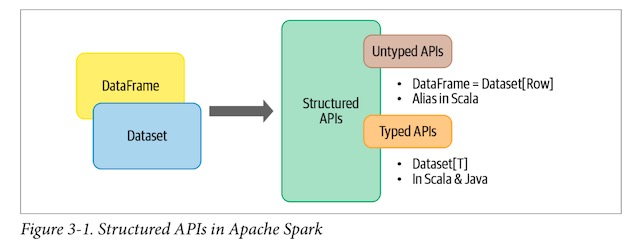### 类型对象、无类型对象和通用的Row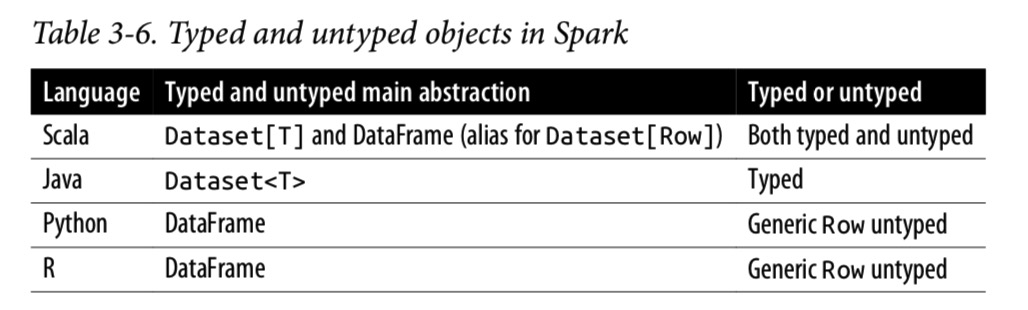### 创建 Dataset

#### Scala： Case classes

JSON file 中每一行大概是这样子：

``````{"device_id": 198164, "device_name": "sensor-pad-198164owomcJZ", "ip":
"80.55.20.25", "cca2": "PL", "cca3": "POL", "cn": "Poland", "latitude":
53.080000, "longitude": 18.620000, "scale": "Celsius", "temp": 21,
"humidity": 65, "battery_level": 8, "c02_level": 1408,"lcd": "red",
"timestamp" :1458081226051}
``````

``````case class DeviceIoTData (battery_level: Long, c02_level: Long, cca2: String, cca3: String, cn: String, device_id: Long, device_name: String, humidity: Long, ip: String, latitude: Double, lcd: String, longitude: Double, scale:String, temp: Long, timestamp: Long)
``````

``````// In Scala
.json("/databricks-datasets/learning-spark-v2/iot-devices/iot_devices.json")
.as[DeviceIoTData]
ds: org.apache.spark.sql.Dataset[DeviceIoTData] = [battery_level...]
ds.show(5, false)
``````

### Dataset 的操作

``````// In Scala
val filterTempDS = ds.filter({d => {d.temp > 30 && d.humidity > 70}) filterTempDS: org.apache.spark.sql.Dataset[DeviceIoTData] = [battery_level...] filterTempDS.show(5, false)
``````

``````// In Scala
case class DeviceTempByCountry(temp: Long, device_name: String, device_id: Long, cca3: String)
val dsTemp = ds
.filter(d => {d.temp > 25})
.map(d => (d.temp, d.device_name, d.device_id, d.cca3))
.toDF("temp", "device_name", "device_id", "cca3")
.as[DeviceTempByCountry]

dsTemp.show(5, false)

val dsTemp2 = ds
.select(\$"temp", \$"device_name", \$"device_id", \$"device_id", \$"cca3")
.where("temp > 25")
.as[DeviceTempByCountry]

``````

## DataFrame VS Dataset

• 如果您想告诉Spark该做什么而不是该怎么做，请使用DataFrames或Datasets。
• 如果您想要丰富的语义，高级抽象和DSL运算符，请使用DataFrames 或 Datasets。
• 如果您希望严格的编译时类型安全性，并且不介意为特定的数据集[T]创建多个case class，请使用dataset。
• 如果您的处理需要高级表达式，过滤器，映射，聚合，计算平均值或总和，SQL查询，列访问或对半结构化数据使用关系运算符，请使用DataFrames或Datasets。
• 如果您的处理要求与类似SQL的查询类似的关系转换，请使用DataFrames。
• 如果您想利用Tungsten的高效编码器序列化并从中受益，请使用Dataset。
• 如果要跨Spark组件进行统一，代码优化和API简化，请使用DataFrames。
• 如果您是R用户，请使用DataFrames。
• 如果您是Python用户，则在需要更多控制权时，请使用DataFrames并下拉至RDD。
• 如果需要空间和速度效率，请使用DataFrames。
• 如果要在编译期间而不是在运行时捕获错误，请选择适当的API，如下图所示：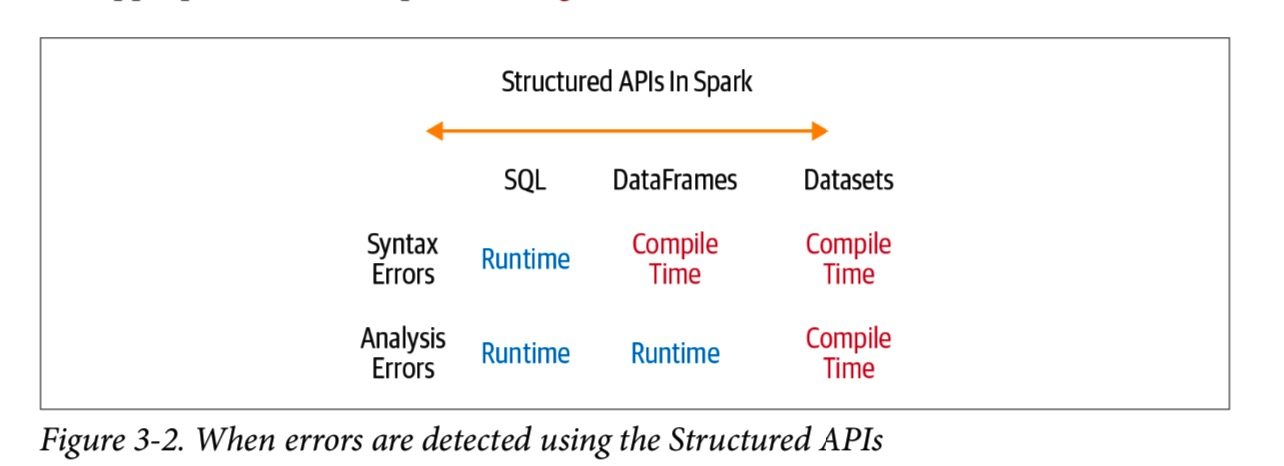### 什么时候使用RDD

• 使用RDD编写的第三方程序包
• 可以放弃代码优化、高效的空间利用以及DataFrame和Dataset所提供的性能优势
• 想精确地指导Spark如何进行查询

## Spark SQL 和底层引擎

Spark 1.3中引入以来，Spark SQL已经发展成为一个强大的引擎，并在其上构建了许多高级结构化功能。 除了允许您对数据发出类似SQL的查询之外，Spark SQL引擎还支持：

• 统一Spark组件，并允许抽象为Java，Scala，Python和R中的DataFrame /dataset，从而简化了结构化数据集的工作。
• 连接到Apache Hive metastore和table。
• 从结构化文件格式（JSON，CSV，文本，Avro，Parquet，ORC等）中以特定模式读取和写入结构化数据，并将数据转换为临时表。
• 提供交互式Spark SQL Shell，以快速浏览数据。
• 通过标准数据库JDBC / ODBC连接器提供与外部工具之间的桥梁。
• 生成用于JVM的优化查询计划和紧凑代码，以最终执行。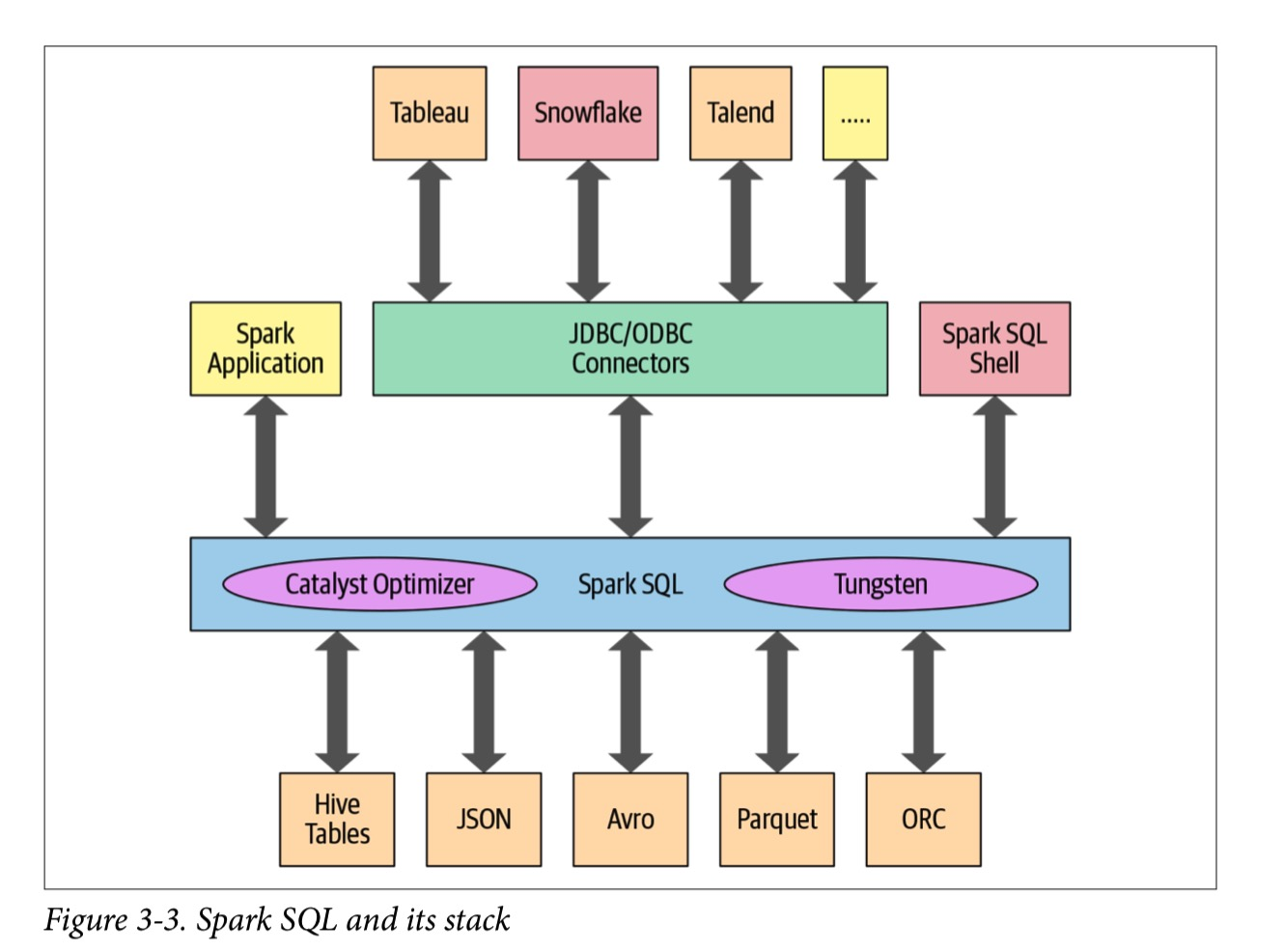Spark SQL引擎的核心是Catalyst优化器和Project Tungsten。 它们一起支持高级DataFrame和Dataset API和SQL查询。 现在，让我们仔细看看优化器。

### The Catalyst Optimizer

Catalyst Optimizer 将可执行的查询转换为一个可执行计划。它通过以下四个阶段：

1. 分析 Analysis
2. 逻辑优化 Logical optimization
3. 物理计划 Physical planning
4. 产生代码 Code generation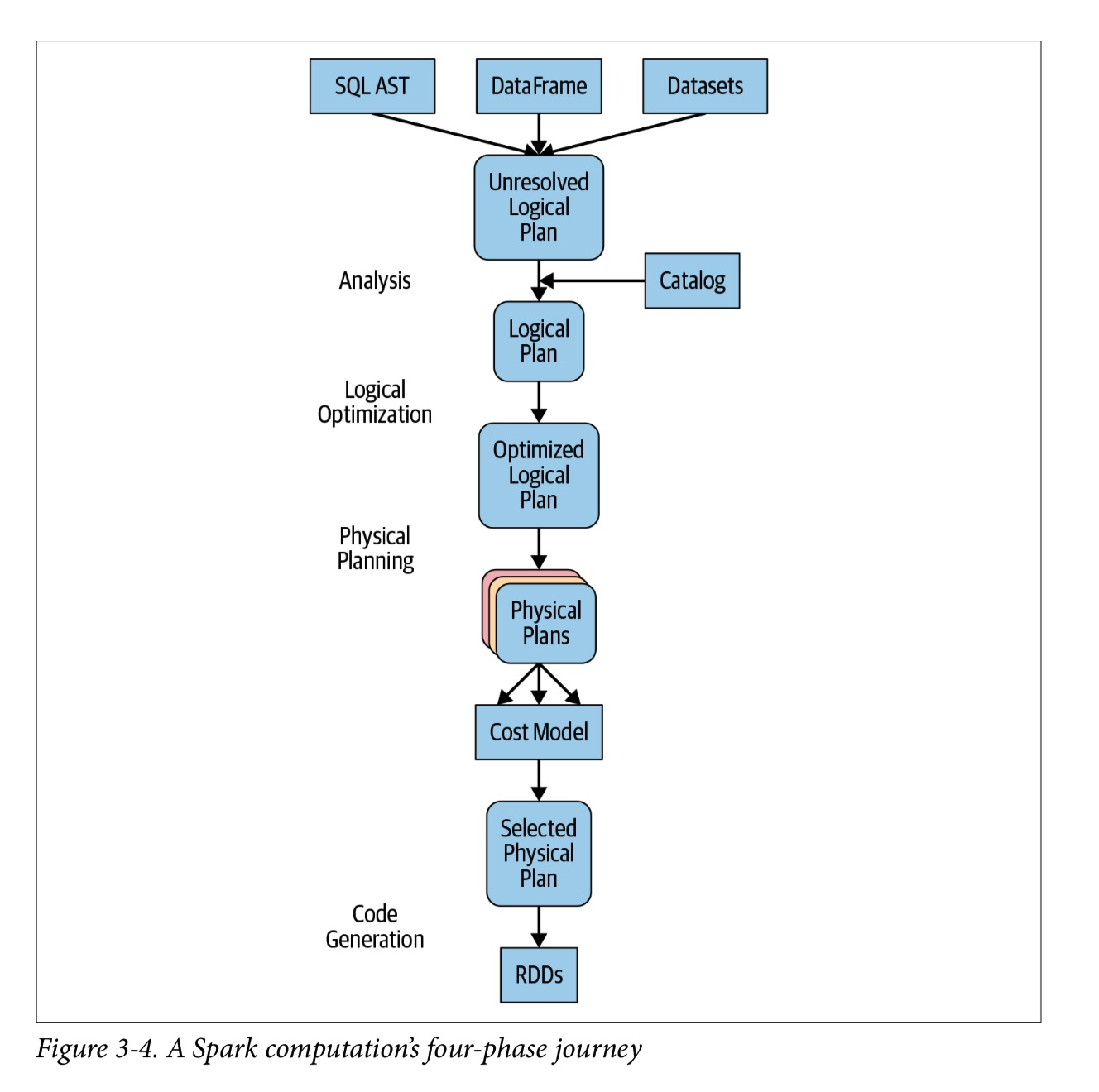``````# In Python
count_mnm_df = (mnm_df
.select("State", "Color", "Count")
.groupBy("State", "Color")
.agg(count("Count").alias("Total"))
.orderBy("Total", ascending=False))
``````
``````-- In SQL
SELECT State, Color, Count, sum(Count) AS Total FROM MNM_TABLE_NAME
GROUP BY State, Color, Count
ORDER BY Total DESC
``````

``````count_mnm_df.explain(True)

== Parsed Logical Plan ==
'Sort ['Total DESC NULLS LAST], true
+- Aggregate [State#10, Color#11], [State#10, Color#11, count(Count#12) AS...]
+- Project [State#10, Color#11, Count#12]
+- Relation[State#10,Color#11,Count#12] csv

== Analyzed Logical Plan ==
State: string, Color: string, Total: bigint
Sort [Total#24L DESC NULLS LAST], true
+- Aggregate [State#10, Color#11], [State#10, Color#11, count(Count#12) AS...]
+- Project [State#10, Color#11, Count#12]
+- Relation[State#10,Color#11,Count#12] csv

== Optimized Logical Plan ==
Sort [Total#24L DESC NULLS LAST], true
+- Aggregate [State#10, Color#11], [State#10, Color#11, count(Count#12) AS...]
+- Relation[State#10,Color#11,Count#12] csv

== Physical Plan ==
*(3) Sort [Total#24L DESC NULLS LAST], true, 0
+- Exchange rangepartitioning(Total#24L DESC NULLS LAST, 200)
+- *(2) HashAggregate(keys=[State#10, Color#11], functions=[count(Count#12)], output=[State#10, Color#11, Total#24L])
+- Exchange hashpartitioning(State#10, Color#11, 200) +- *(1) HashAggregate(keys=[State#10, Color#11],
functions=[partial_count(Count#12)], output=[State#10, Color#11, count#29L]) +- *(1) FileScan csv [State#10,Color#11,Count#12] Batched: false,
Format: CSV, Location: InMemoryFileIndex[file:/Users/jules/gits/LearningSpark2.0/chapter2/py/src/... dataset.csv], PartitionFilters: [], PushedFilters: [], ReadSchema: struct<State:string,Color:string,Count:int>
``````

``````// In Scala
// Users DataFrame read from a Parquet table val usersDF = ...
// Events DataFrame read from a Parquet table val eventsDF = ...
// Join two DataFrames
val joinedDF = users
.join(events, users("id") === events("uid"))
.filter(events("date") > "2015-01-01")
``````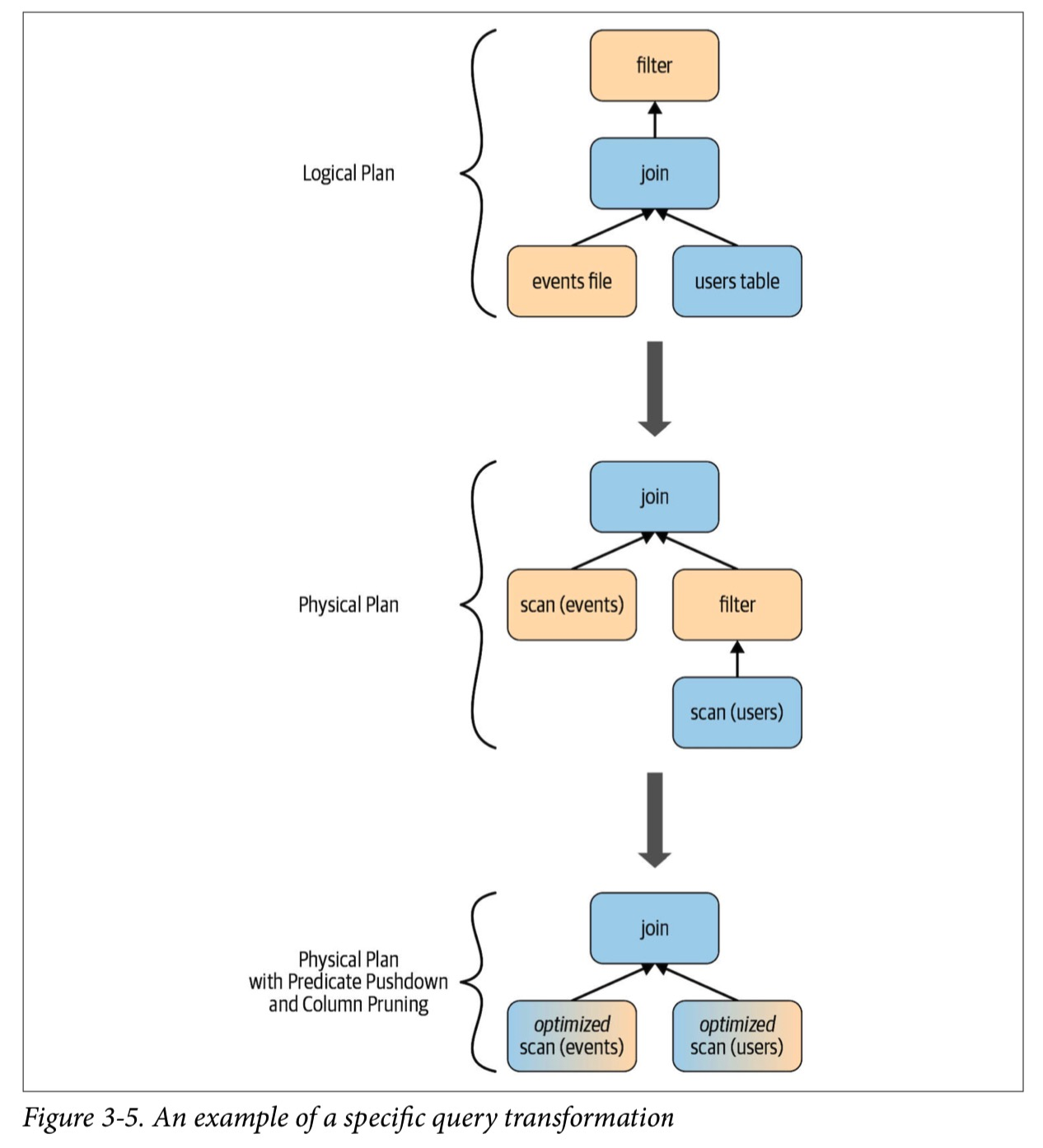#### 阶段1: 分析

Spark SQL引擎首先为SQL或DataFrame查询生成抽象语法树（AST）。 在此初始阶段，将通过查询内部catalog（Spark SQL的编程接口，其中包含列，数据类型，函数，表，数据库等的名称的列表）来解析任何列或表的名称。 成功解决后，查询将进入下一个阶段。

Content